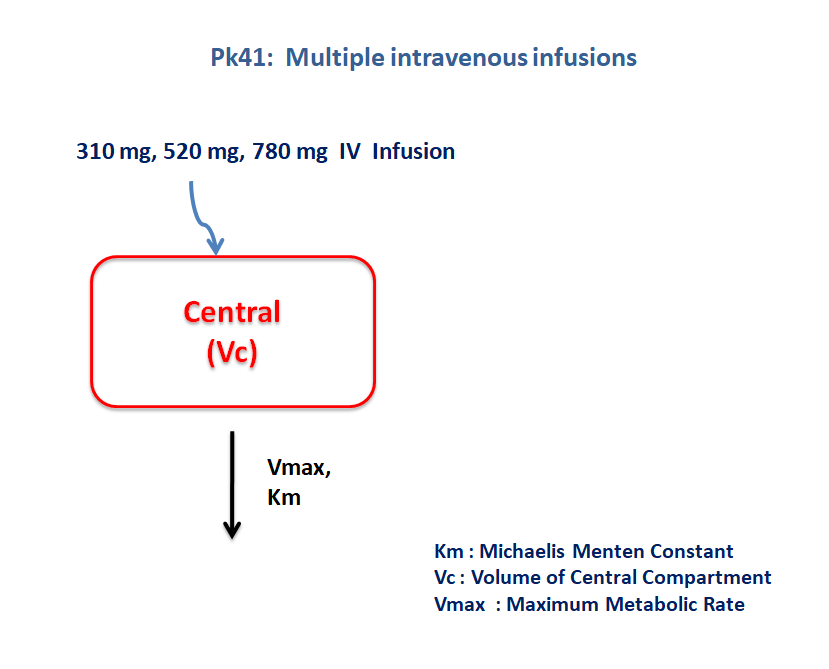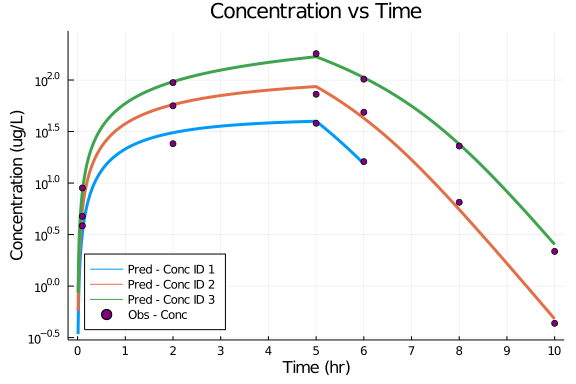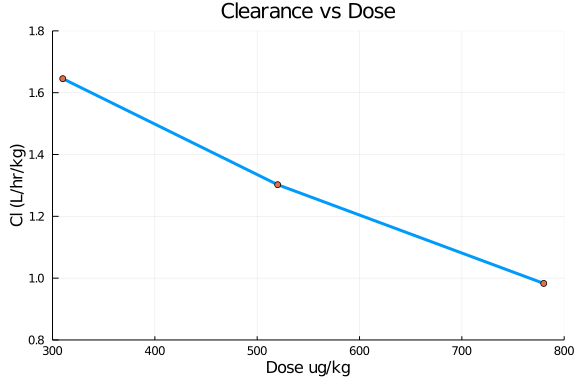# Exercise PK41 - Multiple intraveneous infusions - NCA vs regression

### Background

• Structural Model - One compartment model with non-linear elimination.

• Route of administration - IV infusion

• Dosage Regimen - 310 μg, 520 μg, 780 μg

• Number of Subjects - 3### Learning Outcome

This is a one compartment model with capacity limited elimination. Concentration time profile was obtained for three subjects administered with three different dosage regimens.

### Objectives

In this tutorial, you will learn how to build one compartment model with non-linear elimination.

### Libraries

call the "necessary" libraries to get start.

using Pumas
using Plots
using CSV
using StatsPlots
using Random


### Model

The following model describes the parameters and differential equation for a one-compartment model with capacity limited elimination

pk_41        = @model begin
@param begin
tvvmax   ∈ RealDomain(lower=0)
tvkm     ∈ RealDomain(lower=0)
tvvc     ∈ RealDomain(lower=0)
Ω        ∈ PDiagDomain(3)
σ²_prop  ∈ RealDomain(lower=0)
end

@random begin
η        ~ MvNormal(Ω)
end

@pre begin
Vmax     = tvvmax * exp(η)
Km       = tvkm * exp(η)
Vc       = tvvc  * exp(η)
end

@dynamics begin
Central' = - (Vmax * (Central/Vc)/(Km + (Central/Vc)))
end

@derived begin
cp       = @. Central/Vc
dv       ~ @. Normal(cp, sqrt(cp^2*σ²_prop))
nca     := @nca cp
cl       = NCA.cl(nca)
end
end

PumasModel
Parameters: tvvmax, tvkm, tvvc, Ω, σ²_prop
Random effects: η
Covariates:
Dynamical variables: Central
Derived: cp, dv, cl
Observed: cp, dv, cl


### Parameters

The parameters are as given below. tv represents the typical value for parameters.

• Vmax - Maximum Metabolic Rate (μg/kg/hr)

• Km - Michaelis Menten Constant (μg/kg/L)

• Vc - Volume of Central compartment (L/kg)

param = ( tvvmax  = 180.311,
tvkm    = 79.8382,
tvvc    = 1.80036,
Ω       = Diagonal([0.0,0.0,0.0,0.0,0.0]),
σ²_prop = 0.015)

(tvvmax = 180.311, tvkm = 79.8382, tvvc = 1.80036, Ω = [0.0 0.0 … 0.0 0.0;
0.0 0.0 … 0.0 0.0; … ; 0.0 0.0 … 0.0 0.0; 0.0 0.0 … 0.0 0.0], σ²_prop = 0.0
15)


### Dosage Regimen

• Subject-1 receives a dose of 310 μg given as an IV Infusion over 5 hrs

• Subject-2 receives a dose of 520 μg given as an IV Infusion over 5 hrs

• Subject-3 receives a dose of 780 μg given as an IV Infusion over 5 hrs

ev1      = DosageRegimen(310, cmt=1, time=0, rate=62, route=NCA.IVInfusion)
sub1     = Subject(id=1, events=ev1)
ev2      = DosageRegimen(520, cmt=1, time=0, rate=104, route=NCA.IVInfusion)
sub2     = Subject(id=2, events=ev2)
ev3      = DosageRegimen(780, cmt=1, time=0, rate=156, route=NCA.IVInfusion)
sub3     = Subject(id=3, events=ev3)
pop3_sub = [sub1,sub2,sub3]

Population
Subjects: 3
Covariates:


### Simulation

Simulate the plasma concentration of the drug for both the subjects

Random.seed!(123)
sim_pop3_sub = simobs(pk_41, pop3_sub, param, obstimes=0:0.01:10)
df1          = DataFrame(sim_pop3_sub)


### Dataframe & Plot

Split the simulation to the necessary dataframe and use it for plotting

##### Regression Analysis - Concentration vs Time
df_id1 = filter(x -> x.id == "1", df1)
filter!(x -> x.time <= 6, df_id1)
df_id2 = filter(x -> x.id == "2", df1)
df_id3 = filter(x -> x.id == "3", df1)
df_dv_1 = filter(x -> x.time in [0.1,2,5,6], df_id1)
df_dv_2 = filter(x -> x.time in [0.1,2,5,6,8,10], df_id2)
df_dv_3 = filter(x -> x.time in [0.1,2,5,6,8,10], df_id3)

@df df_id1 plot(:time, :cp, yaxis=:log,
title ="Concentration vs Time", xlabel="Time (hr)", ylabel="Concentration (ug/L)",
label ="Pred - Conc ID 1", linewidth=3, legend=:bottomleft,
xticks=[0,1,2,3,4,5,6,7,8,9,10], xlims=(-0.2,10.2), ylims=(0.3,300))
@df df_id2 plot!(:time, :cp, label ="Pred - Conc ID 2", linewidth=3)
@df df_id3 plot!(:time, :cp, label ="Pred - Conc ID 3", linewidth=3)
@df df_dv_1 scatter!(:time, :dv, label="Obs - Conc", color=[:purple])
@df df_dv_2 scatter!(:time, :dv, label=false, color=[:purple])
@df df_dv_3 scatter!(:time, :dv, label=false, color=[:purple])##### NCA - Clearance vs Dose
df_cl = filter(x -> x.time == 0.1, df1)
df_cl[!, :dose] .= [310,520,780]
select!(df_cl, :dose, :cl)

@df df_cl plot(:dose, :cl,
xlabel="Dose ug/kg", ylabel = "Cl (L/hr/kg)", label=false,
title="Clearance vs Dose", linewidth=3,
xticks=[300,400,500,600,700,800], xlims=(300,800), ylims=(0.8,1.8), yticks=[0.8,1.0,1.2,1.4,1.6,1.8])
@df df_cl scatter!(:dose, :cl, label=false)